# Intermediate Geometry : How to find if parallelograms are similar

## Example Questions

### Example Question #270 : Quadrilaterals

A parallelogram has adjacent sides with the lengths ofand. Find a pair of possible adjacent side lengths for a similar parallelogram.andandandandandExplanation:

Since the two parallelogram are similar, each of the corresponding sides must have the same ratio.

The solution is: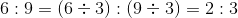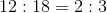, (divide both numbers by the common divisor of).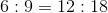### Example Question #1 : How To Find If Parallelograms Are Similar

A parallelogram has adjacent sides with the lengths ofand. Find a pair of possible adjacent side lengths for a similar parallelogram.andandandandandExplanation:

Since the two parallelogram are similar, each of the corresponding sides must have the same ratio.

The ratio of the first parallelogram is:Applying this ratio we are able to find the lengths of a similar parallelogram.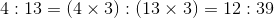### Example Question #2 : How To Find If Parallelograms Are Similar

A parallelogram has adjacent sides with the lengths ofand. Find a pair of possible adjacent side lengths for a similar parallelogram.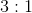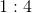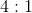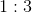Explanation:

Since the two parallelogram are similar, each of the corresponding sides must have the same ratio.

The ratio of the first parallelogram is: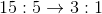Applying this ratio we are able to find the lengths of the second parallelogram.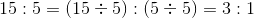### Example Question #3 : How To Find If Parallelograms Are Similar

A parallelogram has adjacent sides with the lengths ofand. Find a pair of possible adjacent side lengths for a similar parallelogram.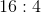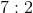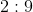Explanation:

Since the two parallelogram are similar, each of the corresponding sides must have the same ratio.

The ratio of the first parallelogram is: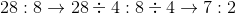Thus by simplifying the ratio we can see the lengths of the similar triangle.